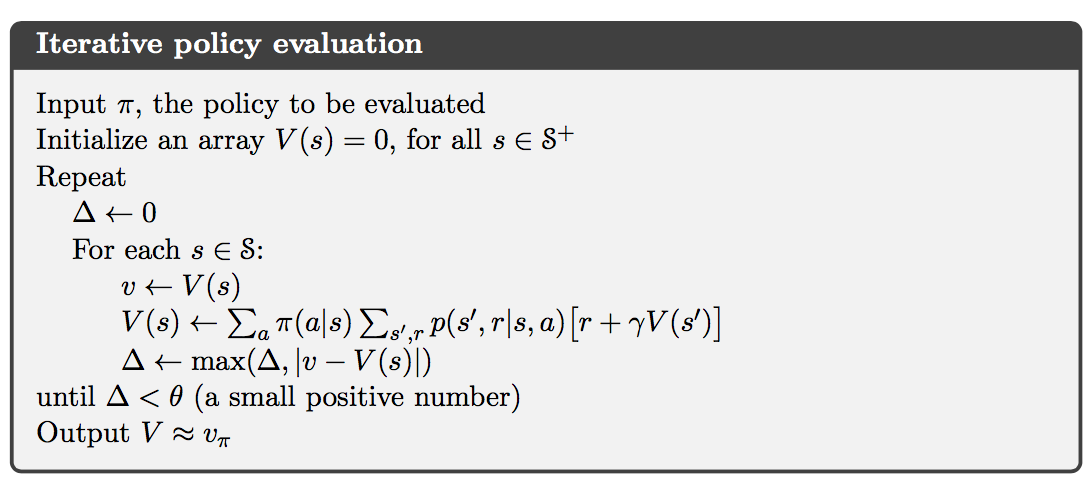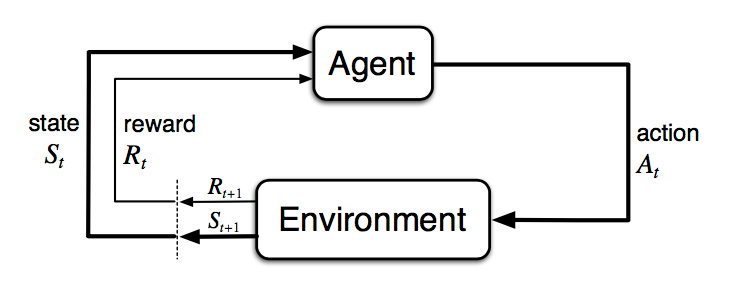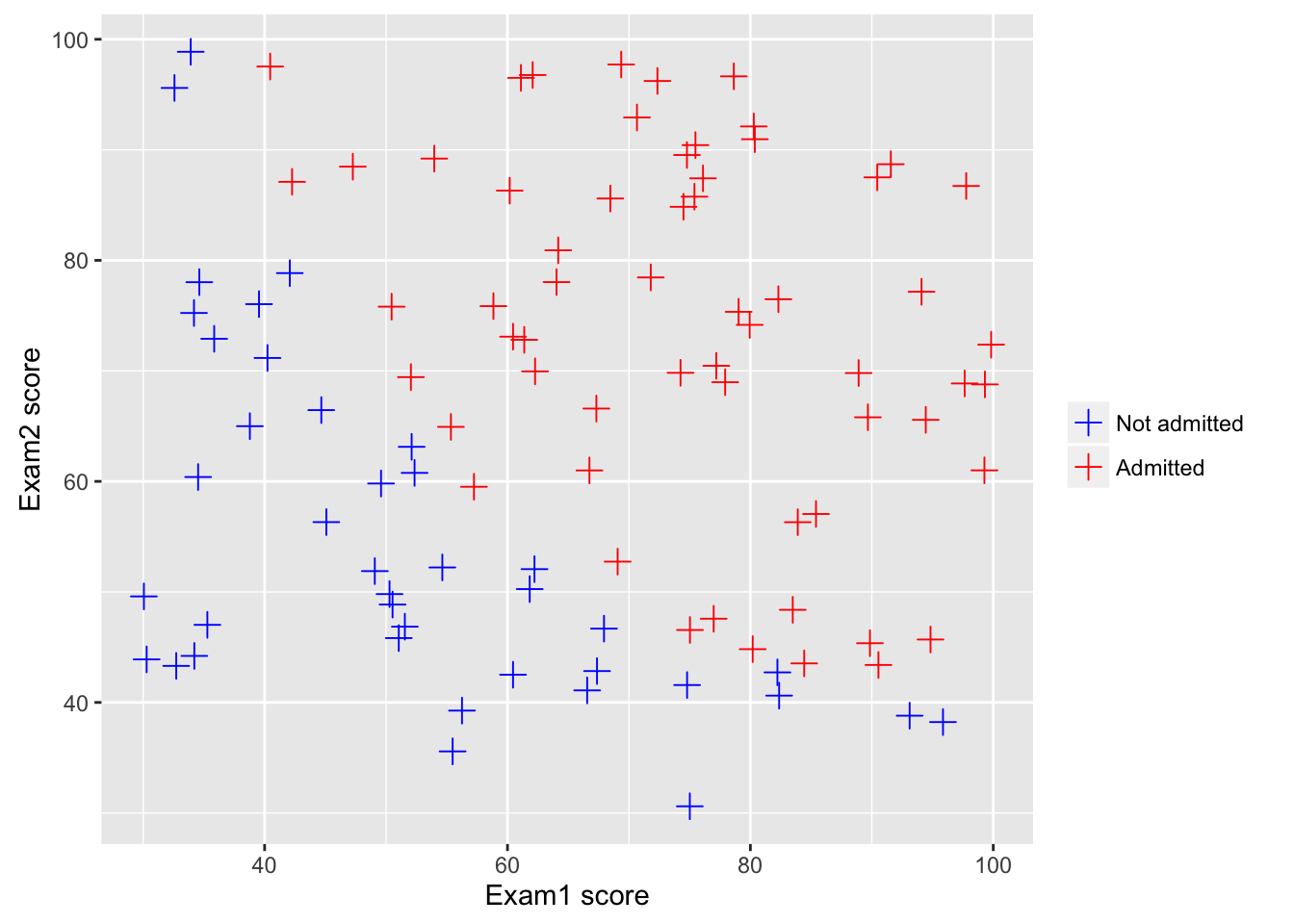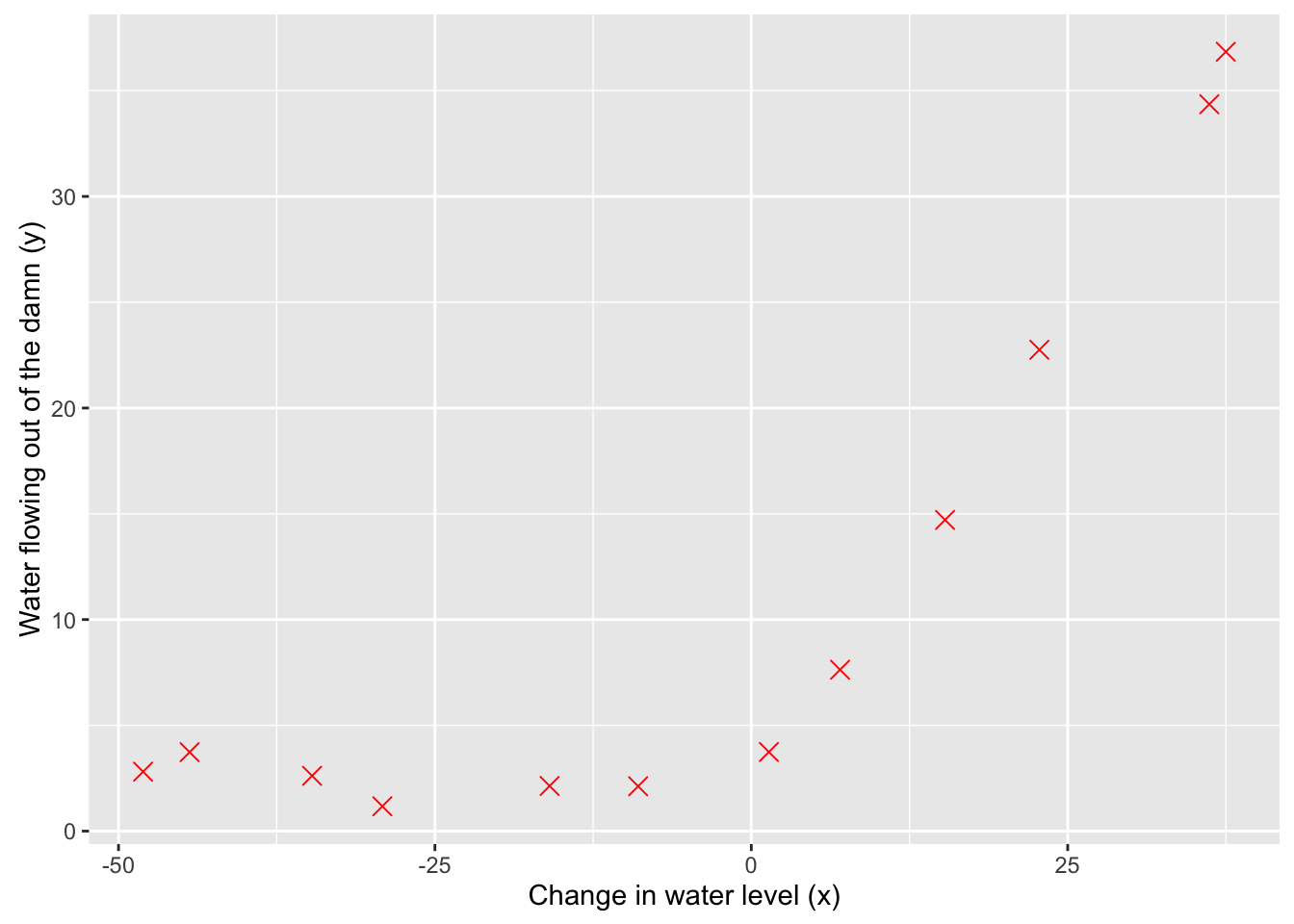# Notes on Reinforcement Learning (2): Dynamic Programming

### Policy Evaluation

Consider a sequence of approximate value functions $v_0, v_1, v_2, \dots,$ each mapping $\mathcal{S}^+$ to $\mathbb{R}$. The initial approximation, $v_0$ is chosen arbitrarily, and each successive approximation is obtained by using the Bellman equation for $v_\pi$ as an update rule:

for all $s\in\mathcal{S}$.# Notes on Reinforcement Learning (1): Finite Markov Decision Processes

### The Agent-Environment Interface• The agent and environment interact at each of a sequence of discrete time steps, $t=0,1,2,3,\dots$
• At each time step $t$, the agent receives some representation of the environment’s state, $S_t\in\mathcal{S}$, where $\mathcal{S}$ is the set of possible states.
• On that basis, the agent selects an action, $A_t \in \mathcal{A}(S_t)$, where $\mathcal{A}(S_t)$ is the set of actions available in state $S_t$.
• One time step later, in part as a consequence of its action, the agent receives a numerical reward, $R_{t+1} \in \mathcal{R} \subset \mathbb{R}$, and finds itself in a new state, $S_{t+1}$.

# ML With R (3): Logistic Regression

Code in R for Coursera-ML and CMPUT466/551 in University of Alberta

This post refers to programming exercise 2 in Coursera-ML. All the code can be found in this repo.

setwd("~/Coding/R/CMPUT466/mlclass-ex2")
library(ggplot2)
library(R.matlab)
library(devtools)
source_url("https://raw.githubusercontent.com/ggrothendieck/gsubfn/master/R/list.R")

### Logistic Regression

#### Visualizing the data

Use a scatter plot to visualize the data.

data <- read.csv("ex2data1.txt", header=FALSE)
X <- as.matrix(data[,c("V1", "V2")])
y <- as.matrix(data$V3) ggplot(data, aes(x=V1, y=V2, col=factor(V3))) + geom_point(shape=I(3), size=I(3)) + labs(x="Exam1 score", y="Exam2 score") + scale_color_manual(name="", labels=c("Not admitted", "Admitted"), values = c("blue", "red"))# ML With R (2): Regularized Linear Regression | Comments Code in R for Coursera-ML and CMPUT466/551 in University of Alberta This post refers to programming exercise 5 in Coursera-ML. All the code can be found in this repo. setwd("~/Coding/R/CMPUT466/mlclass-ex5") library(ggplot2) library(R.matlab) library(devtools) source_url("https://raw.githubusercontent.com/ggrothendieck/gsubfn/master/R/list.R") ### Regularized Linear Regression #### Visualizing the dataset Use a scatter plot to visualize the data. data <- readMat("ex5data1.mat") X <- data$X
y <- data$y Xtest <- data$Xtest
ytest <- data$ytest Xval <- data$Xval
yval <- data\$yval
df <- data.frame(X=X, y=y)
ggplot(df, aes(X, y, col="Training data")) + geom_point(shape=I(4), size=I(3)) +
labs(x="Change in water level (x)", y="Water flowing out of the damn (y)") +
scale_color_manual(guide=FALSE, values=c("Red"))